# speaq 2.0 function illustrations

## speaq 2.0

To illustrate the possibilities of speaq 2.0, the wine dataset is used (available form the University of Copenhagen at models.life.ku.dk). This dataset is also utilized in the paper so with this vignette you will be able to reproduce the results.

Before we start with the example let's first recap what the new method in speaq 2.0 encompass:

1. Peak detection: `getWaveletPeaks()`
2. Peak grouping: `PeakGrouper()`
3. Peak filling: `PeakFilling()`
4. Feature matrix construction: `BuildFeatureMatrix()`

### Plotting the raw wine data

``````library(speaq)
data(Winedata)
Spectra.wine <- as.matrix(Winedata\$spectra )
ppm.wine <- as.numeric(Winedata\$ppm)
wine.color <- Winedata\$wine.color
wine.origin <- Winedata\$origin
# all spectra
speaq::drawSpecPPM(Y.spec = Spectra.wine,
X.ppm = ppm.wine,
title = 'Wine data spectra',
groupFactor = wine.color,
legend.extra.x = 1,
legend.extra.y = 1.1)``````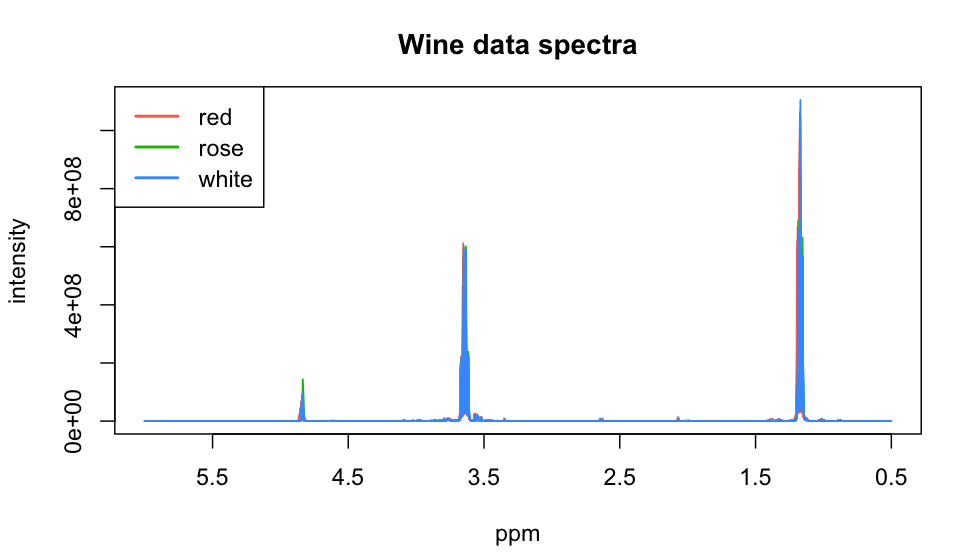The `drawSpecPPM()` plotting function indicates that there might be a gap in the data (which is correct as the original authors deleted the region between 5.0 and 4.5 ppm). This warning can be of importance for the interpretation of the plot as in this case the original authors deleted the data, not by setting the values to 0, but by effectively removing it from the matrix and ppm vector. This produces a plot that appears continuous but is in fact not.

The `drawSpecPPM()` function also indicates that the groupFactor is not a factor and (successfully) attempts to do the conversion. The next plot is an excerpt of the wine NMR spectra.

``````# small excerpt by defining the region of interest
speaq::drawSpecPPM(Y.spec = Spectra.wine,
X.ppm = ppm.wine,
groupFactor = as.factor(wine.color),
title = 'Raw wine data excerpt',
legend.extra.x = 1.1,
legend.extra.y = 1.0,
ROI.ppm = 3.6,
ROI = NULL,
roiWidth.ppm = 0.15,
legendpos = "topright" )``````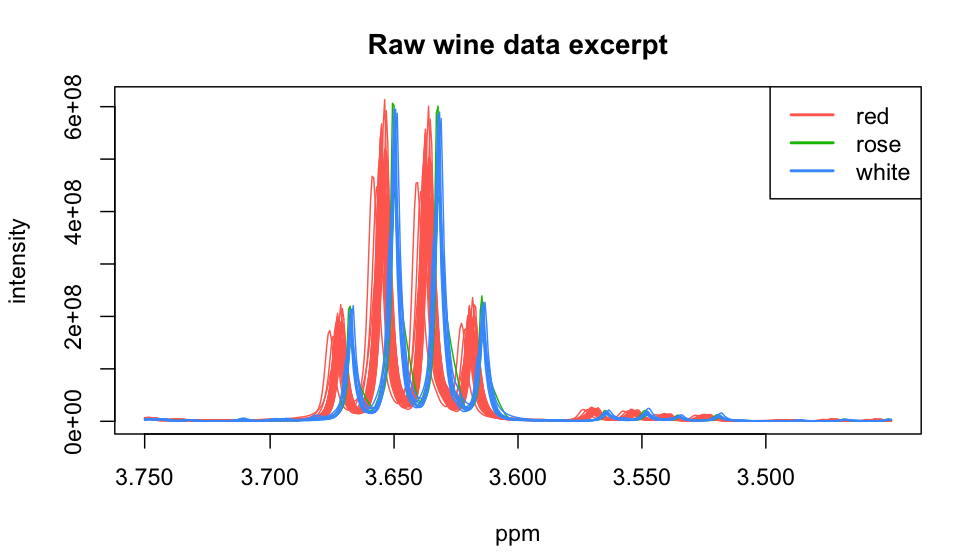### From spectra via peaks to peak groups (features)

Now that we've had a look at the spectra it is time to convert these to peaks by using the `getWaveletPeaks()` function. This takes about 50 seconds, with `nCPU = 4` on a 2.5GHz machine (`nCPU` is set to 1 for the vignette build but should be changed).

``````wine.peaks <- speaq::getWaveletPeaks(Y.spec=Spectra.wine,
X.ppm=ppm.wine,
baselineThresh = 10,
SNR.Th = -1,
nCPU = 2,
include_nearbyPeaks = TRUE) # nCPU set to 2 for the vignette build

wine.grouped <- speaq::PeakGrouper(Y.peaks = wine.peaks,
min.samp.grp = 5,
grouping.window.width = 200)``````

There are two notes to be made on some important parameters: 1. The `include_nearbyPeaks = TRUE` option also detects peaks in the tails of larger other peaks. At first this might seem the preferred option as you want to detect as much peaks as possible but we will see later that often it is better to exclude them as these small peaks can cause problems later on. 2. The `grouping.window.width` parameter is chosen to be twice the size of the default value: it is advised to choose this larger when working with spectra that exhibit large between sample shifts. The wine dataset is an extreme example of large between sample shifts (caused by the substantial pH differences between wines of different colour)

Now we can plot the detected peaks and the grouped peaks. The dataset after grouping contains both the original ppm values of every peak (in the `peakPPM` variable) but also the group information (found in the `peakIndex` variable). By calling the `AddPlottingStuff()` function the groupPPM variable is added so we also have the ppm value of the groups (the link is: `groupPPM <- ppm.wine[peakIndex]`)

### Plotting the peak data

``````# adding labels to the dat a for plotting and the group ppm values
library(ggplot2)
ROI.ppm <- 1.330
roiWidth.ppm <- 0.025

speaq::ROIplot(Y.spec = Spectra.wine,
X.ppm = ppm.wine,
ungrouped.peaks = wine.peaks,
grouped.peaks = wine.grouped,
ROI.ppm = ROI.ppm,
roiWidth.ppm = roiWidth.ppm,
groupLabels = as.factor(wine.color))``````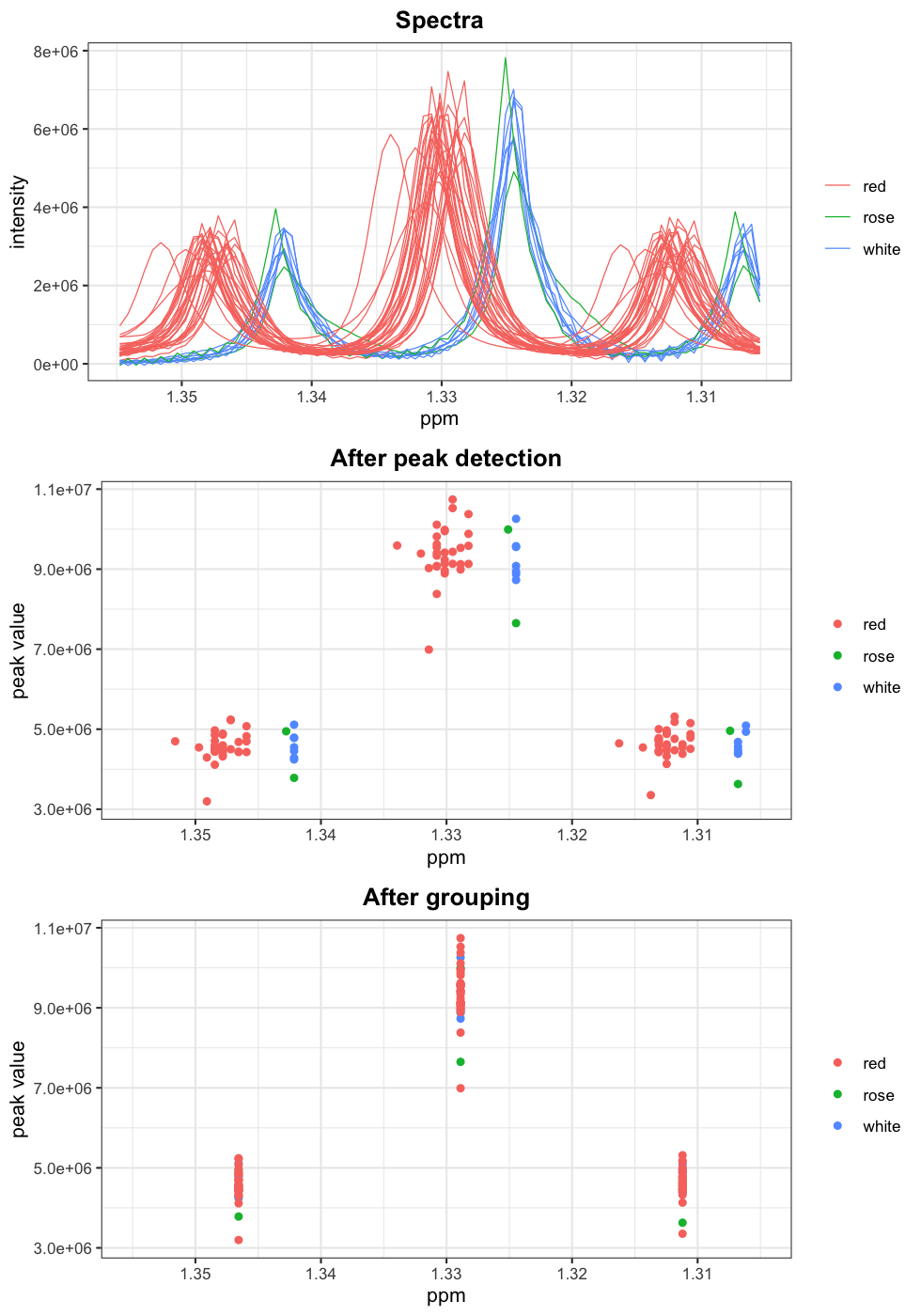The plot above shows clearly what the basis of speaq 2.0 does, convert spectra accurately to peak data and group these peaks. The quality of the grouping can be checked by plotting the silhouette values. this can be done with the `SilhouetR()` function.

``````SilhouetteValues <- speaq::SilhouetR(DataMatrix = wine.grouped\$peakPPM,
GroupIndices = wine.grouped\$peakIndex)``````
``## DataMatrix is not a matrix, attempting conversion with the assumption of only 1 variable (1 column)``
``````Silh_plot <- ggplot(SilhouetteValues, aes(SilhouetteValues)) +
geom_freqpoly(binwidth = 0.03) +
theme_bw()
Silh_plot``````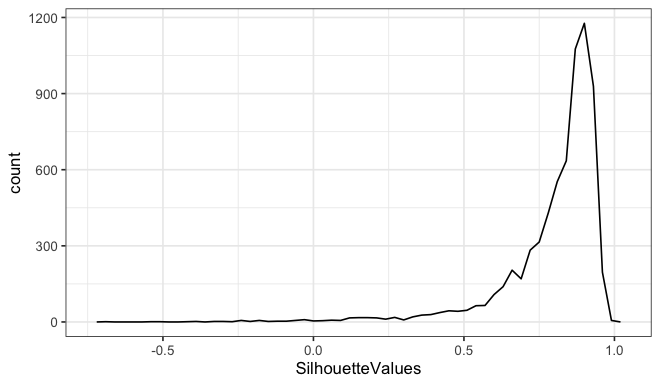It is clear that the grouping is very good. To be absolutely sure we can check the mean silhouette value of every group to see if there are groups that should be regrouped. This regrouping, if needed, can be done with the `regroupR()` function.

``````groups <- unique(SilhouetteValues\$GroupIndices)
Ngroups <- length(groups)
sil_means <- matrix(NA, ncol = 3, nrow = Ngroups)

for(k in 1:Ngroups){
sil_means[k,1] = groups[k]
sil_means[k,2] = mean(SilhouetteValues\$SilhouetteValues[SilhouetteValues\$GroupIndices==groups[k]])
sil_means[k,3] = mean(wine.grouped\$peakSNR[wine.grouped\$peakIndex==groups[k]])
}

sil_means <- sil_means[order(sil_means[,2]),]
colnames(sil_means) <- c("groupIndex", "avg_silhouette_val", "avg. SNR")
``````##      groupIndex avg_silhouette_val  avg. SNR
## [1,]       5313          0.2557844 32.261401
## [2,]       5050          0.4549954 11.669748
## [3,]       2330          0.4848325 31.757387
## [4,]       4711          0.4904269  5.754990
## [5,]       5552          0.5011936  6.933832
## [6,]       3287          0.5099114  3.559727``````

As it turns out, there is a group with a low average silhouette value (0.25) but a fairly high average signal-to-noise ratio (if this group had a low SNR ratio it could just be a noise group). This indicates that these are non-noise peaks which are grouped incorrectly, the following plot acknowledges this

``````faulty.groupIndex <- sil_means[1,1]
ROI.ppm <- ppm.wine[faulty.groupIndex]
roiWidth.ppm <- 0.1

speaq::ROIplot(Y.spec = Spectra.wine,
X.ppm = ppm.wine,
ungrouped.peaks = wine.peaks,
grouped.peaks = wine.grouped,
ROI.ppm = ROI.ppm,
roiWidth.ppm = roiWidth.ppm,
groupLabels = as.factor(wine.color))``````
``## Warning: Removed 2 rows containing missing values (geom_point).``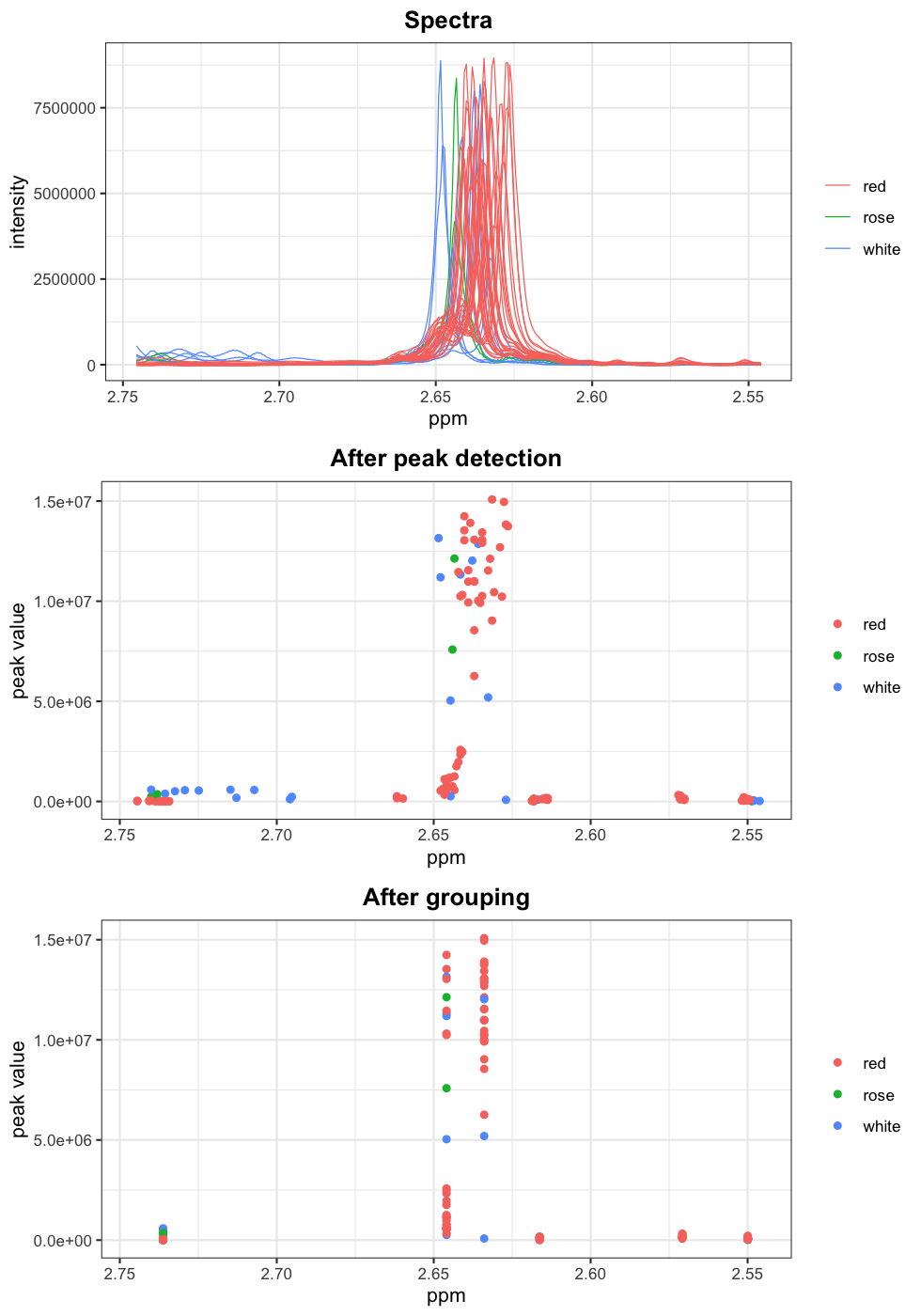Clearly the large peaks and small peaks are grouped together because of the large shifts in the wine dataset making the small and large peaks overlap, this messes up the grouping. There are multiple ways to solve this issue:

1. By setting `include_nearbyPeaks = FALSE` in the `getWaveletPeaks()` function these small peaks in the tails of large peaks will not be included, although some other peaks might also be missed. Or

2. by selecting the wrong group and submitting them to the `regroupR()` function. This will use both the ppm values and the peak signal-to-noise ratio for the initial between peak distance calculation and group them according to this distance.

We will use option 2 in this case to demonstrate the use of the `regroupR()` function. Since the wrong grouping of these two overlapping groups also causes a clear misgrouping of the next group we will submit the 3 peak groups (see right half of bottom plot) to the `regroupR()` function to fix the issues.

``````wrong.groups <- sort(sil_means[sil_means[,1]>=sil_means[1,1],1])[1:2]

wine.regrouped <- speaq::regroupR(grouped.peaks = wine.grouped,
list.to.regroup = wrong.groups,
min.samp.grp = 5,
max.dupli.prop = 0.1)``````
``## 'list.to.regroup' is not a list. Assuming it is a list with only one element.``

The plot below reveals the problem is clearly fixed now, but it requires more user interaction than wanted.

``````faulty.groupIndex <- sil_means[1,1]
ROI.ppm <- ppm.wine[faulty.groupIndex]
roiWidth.ppm <- 0.1

speaq::ROIplot(Y.spec = Spectra.wine,
X.ppm = ppm.wine,
ungrouped.peaks = wine.peaks,
grouped.peaks = wine.regrouped,
ROI.ppm = ROI.ppm,
roiWidth.ppm = roiWidth.ppm,
groupLabels = as.factor(wine.color))``````
``## Warning: Removed 2 rows containing missing values (geom_point).``